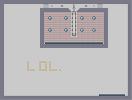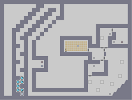### Death PitHover over the thumbnail for a full-size version.

Author xXSc3n1cXx action author:xxsc3n1cxx fun rated 2009-09-02 2009-09-02 3 by 5 people. \$Death Pit#xXSc3n1cXx#action#00000000000000000000000000000000000000000000000000000000000000000000000000000000000000000000000000000000000000000000000000000000000000000000000000000000000000000000000000000000000000000000000000000000000000001111111111000000000000010000000010000000000000100000000100000000000001000000001000000000000010000000010000000000000100000000100000000000001000000001000000000000020000000010000000000000000000000100000000000003000000001000000000000010000000010000000000000100000000100000000000001000000001000000000000010000000010000000000000100000000100000000000001000000001000000000000011111111110000000000000000000000000000000000000000000000000000000000000000000000000000000000000000000000000000000000000000000000000000000|5^444,36!12^384,72!12^360,72!12^372,72!12^372,84!12^384,84!12^360,84!12^360,96!12^372,96!12^384,96!12^384,108!12^372,108!12^360,108!12^360,120!12^360,120!12^372,120!12^384,120!12^384,132!12^372,132!12^360,132!12^360,144!12^372,144!12^384,144!12^384,156!12^372,156!12^360,156!12^348,72!12^336,72!12^324,72!12^324,84!12^336,84!12^348,84!12^348,96!12^348,96!12^348,108!12^348,120!12^348,132!12^348,144!12^348,156!12^336,156!12^336,144!12^336,132!12^336,132!12^336,120!12^336,108!12^336,96!12^324,96!12^324,96!12^324,108!12^324,120!12^324,132!12^324,144!12^324,144!12^324,156!12^312,156!12^312,144!12^312,144!12^312,132!12^312,120!12^312,108!12^312,108!12^312,96!12^312,96!12^312,84!12^312,84!12^312,72!12^300,72!12^300,84!12^300,84!12^300,96!12^300,108!12^300,120!12^300,132!12^300,144!12^300,156!12^300,156!12^288,144!12^288,132!12^288,120!12^288,108!12^288,96!12^288,84!12^288,84!12^288,72!12^276,72!12^276,96!12^276,108!12^276,108!12^276,120!12^276,120!12^276,84!12^276,84!12^276,132!12^276,132!12^276,144!12^276,144!12^288,144!12^288,156!12^276,156!12^276,156!12^276,168!12^276,180!12^276,192!12^276,192!12^276,192!12^276,204!12^276,204!12^276,204!12^276,216!12^288,168!12^300,168!12^312,168!12^324,168!12^336,168!12^348,168!12^360,168!12^372,168!12^384,168!12^384,180!12^372,180!12^360,180!12^348,180!12^336,180!12^312,180!12^312,180!12^300,180!12^288,180!12^288,192!12^300,192!12^312,192!12^324,180!12^324,192!12^336,192!12^348,192!12^360,192!12^360,192!12^372,192!12^384,192!12^384,192!12^396,180!12^396,168!12^396,168!12^396,144!12^396,144!12^396,132!12^396,120!12^396,108!12^396,96!12^396,156!12^396,156!12^396,72!12^408,72!12^408,72!12^408,108!12^408,108!12^408,120!12^408,120!12^408,132!12^408,144!12^408,144!12^408,156!12^408,168!12^408,168!12^408,180!12^408,192!12^396,192!12^384,204!12^396,204!12^408,204!12^372,204!12^360,204!12^336,204!12^324,204!12^312,204!12^288,204!12^312,204!12^300,216!12^300,204!12^288,216!12^324,216!12^324,216!12^336,216!12^348,216!12^348,204!12^372,216!12^372,216!12^384,216!12^396,216!12^408,216!12^348,204!12^360,204!12^360,216!12^312,216!12^312,216!12^468,72!12^468,84!12^480,84!12^480,72!12^492,72!12^480,84!12^492,84!12^504,84!12^504,84!12^504,84!12^504,84!12^516,84!12^516,84!12^516,84!12^516,84!12^516,84!12^516,72!12^504,72!12^468,96!12^468,96!12^468,96!12^468,108!12^468,120!12^468,120!12^468,132!12^468,144!12^468,156!12^468,168!12^468,180!12^468,192!12^468,204!12^468,216!12^468,216!12^480,216!12^480,204!12^480,192!12^480,192!12^480,180!12^480,168!12^480,144!12^480,144!12^480,132!12^480,120!12^480,108!12^480,108!12^480,96!12^492,96!12^492,96!12^504,96!12^504,96!12^516,96!12^516,96!12^516,108!12^516,108!12^516,120!12^504,108!12^504,108!12^492,108!12^492,120!12^504,120!12^516,144!12^516,144!12^504,144!12^504,144!12^492,132!12^516,156!12^504,168!12^504,168!12^492,180!12^504,192!12^480,192!12^492,192!12^492,204!12^492,216!12^504,216!12^504,204!12^504,204!12^516,204!12^516,216!12^528,72!12^528,72!12^540,72!12^552,72!12^552,72!12^564,72!12^564,72!12^576,72!12^576,72!12^588,72!12^588,204!12^540,96!12^540,96!12^528,84!12^528,84!12^540,84!12^552,84!12^564,84!12^564,84!12^564,84!12^576,84!12^588,84!12^588,84!12^588,96!12^588,108!12^588,120!12^588,132!12^588,132!12^588,144!12^588,168!12^588,168!12^588,180!12^588,192!12^588,156!12^588,216!12^576,216!12^564,216!12^552,216!12^552,216!12^528,216!12^540,216!12^540,204!12^528,204!12^528,204!12^528,192!12^528,168!12^528,168!12^528,156!12^528,156!12^528,144!12^528,120!12^528,120!12^528,108!12^528,96!12^552,96!12^552,96!12^564,96!12^576,96!12^576,96!12^576,120!12^576,120!12^576,132!12^576,132!12^576,132!12^576,144!12^576,156!12^576,168!12^576,192!12^576,192!12^576,204!12^576,204!12^552,204!12^552,204!12^564,204!12^540,192!12^540,180!12^540,168!12^552,168!12^552,168!12^552,156!12^540,156!12^540,144!12^540,144!12^552,144!12^540,120!12^552,120!12^552,120!12^576,108!12^552,108!12^420,72!12^420,84!12^408,84!12^396,84!12^408,96!12^420,96!12^420,216!12^420,204!12^420,192!12^420,180!12^420,168!12^420,144!12^420,132!12^420,144!12^420,120!12^420,108!12^420,108!12^600,72!12^612,72!12^612,84!12^612,84!12^600,84!12^600,108!12^600,108!12^600,108!12^600,120!12^600,132!12^600,144!12^600,156!12^600,180!12^600,192!12^600,204!12^600,204!12^600,216!12^612,204!12^612,216!12^612,204!12^612,192!12^612,192!12^612,168!12^600,168!12^612,180!12^612,156!12^612,156!12^612,144!12^612,132!12^612,120!12^612,108!12^612,96!12^600,96!12^420,156!12^420,228!12^444,228!12^456,228!12^432,228!12^468,228!12^480,228!12^492,228!12^504,228!12^492,240!12^480,240!12^468,240!12^456,240!12^444,240!12^420,240!12^408,240!12^408,240!12^432,240!12^396,240!12^408,228!12^384,228!12^372,228!12^396,228!12^384,228!12^372,228!12^348,228!12^336,228!12^312,228!12^300,228!12^288,228!12^288,228!12^276,228!12^276,240!12^288,240!12^312,240!12^312,240!12^324,228!12^324,228!12^324,240!12^288,240!12^300,240!12^348,240!12^360,228!12^360,228!12^360,240!12^336,240!12^336,240!12^360,240!12^372,240!12^372,240!12^384,240!12^516,228!12^528,228!12^528,228!12^540,228!12^564,228!12^564,228!12^576,228!12^576,228!12^576,228!12^588,228!12^600,228!12^600,228!12^600,228!12^612,228!12^612,240!12^600,240!12^588,240!12^576,240!12^564,240!12^552,240!12^552,228!12^540,240!12^528,240!12^516,240!12^504,240!12^612,252!12^600,252!12^600,252!12^588,252!12^576,252!12^564,252!12^540,252!12^528,252!12^504,252!12^480,252!12^468,252!12^456,252!12^432,252!12^432,252!12^444,252!12^420,252!12^408,252!12^396,252!12^396,252!12^384,252!12^372,252!12^360,252!12^348,252!12^336,252!12^324,252!12^312,252!12^312,252!12^300,252!12^288,252!12^288,252!12^276,252!12^528,252!12^540,252!12^552,252!12^516,252!12^492,252!12^528,252!12^516,240!12^528,216!12^528,192!12^528,168!12^540,156!12^528,168!12^528,168!12^516,156!12^408,204!12^384,180!12^360,144!12^348,132!12^348,144!12^336,156!12^348,168!12^324,192!12^312,192!12^360,108!12^360,120!12^372,156!12^360,192!12^360,192!12^348,180!12^348,156!12^348,156!12^348,156!12^348,144!12^348,144!12^348,144!12^348,144!12^348,144!12^348,144!12^348,144!12^348,144!12^348,144!12^348,144!12^348,144!12^348,144!12^348,144!12^348,144!12^348,144!12^348,144!12^348,144!12^348,144!12^348,144!12^348,144!12^348,144!11^600,36,516,36!11^276,36,372,36!4^744,570,1!4^732,570,1!4^708,570,1!4^684,570,1!4^660,570,1!4^696,570,1!4^720,570,1!4^672,570,1!4^648,570,1!4^648,570,1!4^636,570,1!4^624,570,1!4^612,570,1!4^600,570,1!4^600,570,1!4^612,258,1!4^588,258,1!4^588,258,1!4^588,258,1!4^600,258,1!4^576,258,1!4^564,258,1!4^540,258,1!4^540,258,1!4^552,258,1!4^528,258,1!4^504,258,1!4^492,258,1!4^456,258,1!4^504,258,1!4^516,258,1!4^480,258,1!4^468,258,1!4^444,258,1!4^420,258,1!4^420,258,1!4^420,258,1!4^432,258,1!4^396,258,1!4^408,258,1!4^384,258,1!4^360,258,1!4^360,258,1!4^372,258,1!4^348,258,1!4^336,258,1!4^324,258,1!4^312,258,1!4^300,258,1!4^300,258,1!4^288,258,1!4^276,258,1!6^396,108,5,0,0,1!6^300,108,5,0,0,1!6^300,180,5,0,0,1!6^396,180,5,0,0,1!6^564,180,5,0,0,1!6^564,108,5,0,0,1!6^492,108,5,0,0,1!6^492,180,5,0,0,1!12^492,156!12^492,156!12^480,156!12^492,144!12^504,156!12^492,168!12^504,168!12^504,180!12^516,180!12^516,192!12^552,192!12^564,192!12^576,180!12^552,180!12^528,180!12^516,168!12^492,120!12^540,108!12^540,132!12^540,120!12^564,120!12^564,120!12^564,132!12^564,132!12^540,132!12^552,132!12^552,132!12^528,132!12^516,132!12^504,132!12^552,144!12^552,144!12^564,144!12^564,144!12^564,156!12^564,156!12^564,168!12^264,72!12^264,84!12^264,84!12^264,84!12^264,96!12^264,96!12^264,108!12^264,108!12^264,120!12^264,132!12^264,132!12^264,132!12^264,144!12^264,156!12^264,156!12^264,168!12^264,168!12^264,180!12^264,180!12^264,192!12^264,192!12^264,204!12^264,204!12^264,204!12^264,216!12^264,228!12^264,228!12^264,240!12^264,240!12^264,252!12^612,240!12^624,252!12^624,240!12^624,240!12^624,228!12^624,216!12^624,216!12^624,204!12^624,192!12^624,180!12^624,168!12^624,156!12^624,156!12^624,144!12^624,132!12^624,108!12^624,108!12^624,96!12^624,84!12^624,84!12^624,72!12^624,120!7^444,60,3!0^168,372!0^168,384!0^168,396!0^168,408!0^168,420!0^180,420!0^192,420!0^240,372!0^252,372!0^276,372!0^276,384!0^264,372!0^276,396!0^276,408!0^276,408!0^264,420!0^276,420!0^252,420!0^240,420!0^240,408!0^240,396!0^240,384!0^300,372!0^300,384!0^300,396!0^300,408!0^300,420!0^312,420!0^312,420!0^336,420!0^324,420!0^360,420!0^204,420!9^444,60,1,0,18,2,1,0,0!9^444,204,0,0,18,3,1,-1,0!9^444,204,0,0,18,3,1,0,0!9^444,204,0,0,18,4,1,-1,0!9^444,204,0,0,18,4,1,0,0!9^444,204,0,0,18,5,1,-1,0!9^444,204,0,0,18,5,1,0,0!9^444,204,0,0,18,6,1,-1,0!9^444,204,0,0,18,6,1,0,0!9^444,204,0,0,18,7,1,-1,0!9^444,204,0,0,18,7,1,0,0!9^444,204,0,0,18,8,1,-1,0!9^444,204,0,0,18,8,1,0,0!9^444,204,1,0,18,8,1,0,0!9^444,84,1,1,18,3,0,0,-1!9^564,36,0,1,22,1,0,-1,0!9^312,36,0,1,14,1,0,0,0# You Can Die, Or Live. But your choice, changable, it is not.

## Other maps by this authorThe Blue things are coming!